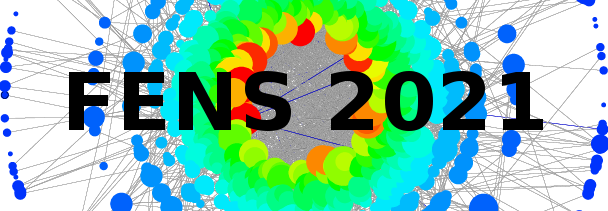#11th Polish Symposium on Physics in Economy and Social Sciences

1-3 July 2021
ONLINE
Europe/Warsaw timezone

## Biased voter model: How persuasive a small group can be?

3 Jul 2021, 10:40
20m
ONLINE

#### ONLINE

Contributed talk (20 min)

### Speaker

Christos Charalambous

### Description

We study the voter model dynamics in the presence of confidence and bias. We assume two types of voters. Unbiased voters (UV) whose confidence is indifferent to the state of the voter and biased voters (BV) whose confidence is biased towards a common fixed preferred state. We study the problem analytically on the complete graph using mean field theory and on a random network topology using the pair approximation, where we assume that the network topology is independent of the type of voters. We verify our analytical results through numerical simulations. We find that for the case of a random initial setup, and for sufficiently large number of voters N, the time to consensus increases proportionally to log(N)/γv, with γ the fraction of biased voters and v the bias of the voters. Finally, we study this model on a biased-dependent topology. We examine two distinct, global average-degree preserving strategies to obtain such biased-dependent random topologies starting from the biased-independent random topology case as the initial setup. We find that increasing the average number of links among only biased voters (BV-BV) at the expense of that of only unbiased voters (UV-UV), while keeping the average number of links among the two types (BV-UV) constant, resulted in a significant decrease in the average time to consensus to the preferred state in the group. Hence, persuasiveness of the biased group depends on how well its members are connected among each other, compared to how well the members of the unbiased group are connected among each other.

### Primary authors

Prof. Raul Toral (IFISC) Prof. Maxi San Miguel (IFISC)

### Presentation Materials

There are no materials yet.